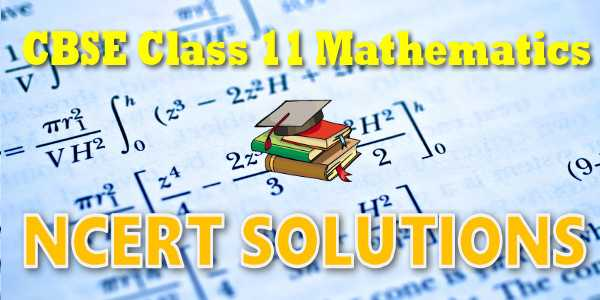# NCERT solutions for class 11 Mathematics## NEET-UG 2021

10,000+ Daily Practice Questions

(only at ₹ 299/- in myCBSEguide App)

## NCERT solutions for class 11 Mathematics## myCBSEguide App

Complete Guide for CBSE Students

NCERT Solutions, NCERT Exemplars, Revison Notes, Free Videos, CBSE Papers, MCQ Tests & more.## NCERT Solutions Chapter 05: Complex Numbers and Quadratic Equations

In earlier classes, we have studied linear equations in one and two variables and quadratic equatiions in one variable. We have seen that the equation x2+1=0 has no real solution as x2+1=0 gives x2=-1 and square of every real number is non-negaive. So, we need to extend the real number system to a larger system so that we can find the solution of the equation x2=-1. In fact, the main objective is to solve the equation ax2+bx+c=0, where D=b2-4ac<0, which is not possible in the system of real numbers.

## NCERT Solutions for Class 11th Mathematics

NCERT Solutions Class 11 Mathematics PDF (Download) Free from myCBSEguide app and myCBSEguide website. Ncert solution class 11 Mathematics includes text book solutions. NCERT Solutions for CBSE Class 11 maths have total 16 chapters. Class 11 Mathematics ncert Solutions in pdf for free Download are given in this website. Ncert Mathematics class 11 solutions PDF and Mathematics ncert class 11 PDF solutions with latest modifications and as per the latest CBSE syllabus are only available in myCBSEguide.

## NCERT Solutions for Class 11## myCBSEguide

Trusted by 1 Crore+ Students

#### CBSE Test Generator

Create papers in minutes

Print with your name & Logo

3 Lakhs+ Questions

Solutions Included

Based on CBSE Blueprint

Best fit for Schools & Tutors

#### Work from Home

• Work from home with us
• Create questions or review them from home

No software required, no contract to sign. Simply apply as teacher, take eligibility test and start working with us. Required desktop or laptop with internet connection